SYLLABUS  Previous: 1.4.5 Harmonic functions  Up: 1.4 Numerical discretization  Next: 1.4.7 Sampling with quasi-particles

### 1.4.6 Wavelets

Starting with a coarse (global) approximation, the idea behind wavelets is to successively refine the representation and store the difference from one scale to the next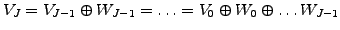(1.4.6#eq.1)

This is best illustrated with Haar wavelets in figure (1.4.6#fig.1), showing that the piecewise constant approximation at a level V4 can be brought to the higher level V5 by adding a correction W4.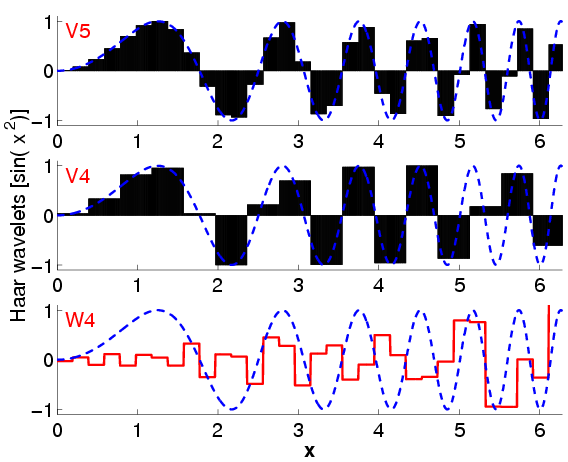Appropriate for integral equations and best suited for the understanding, Haar wavelets are however not practical for the evaluation of derivatives in PDEs. In the spirit of the FFT, Mrs Daubechies proposed a fast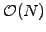linear discrete wavelet transformation (DWT) called DAUB4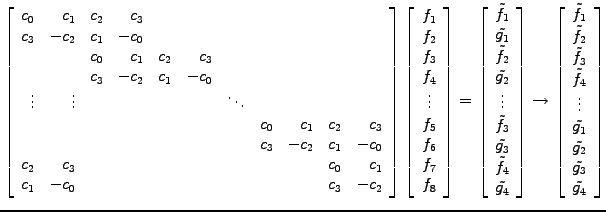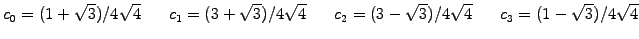(1.4.6#eq.2)

which is applied successively to the function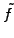together with the permutation until only the first two components remain. The inverse is calculated by reversing the procedure and using the inverse matrix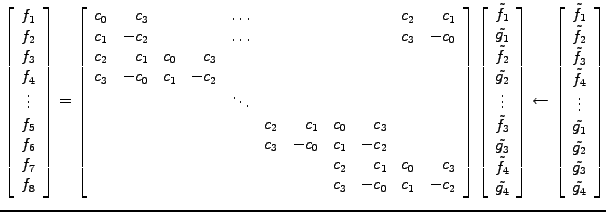(1.4.6#eq.3)

Approximations with DAUB4 have the peculiar property that the derivative exists almost everywhere. Even if wavelets are not smooth, they still can represent exactly piecewise polynomial functions of arbitrary slope - the cusps in the wavelets cancel out exactly! Figure (1.4.6#fig.2) illustrates how the solution converges when the first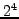DWT components of DAUB4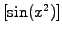are taken to a higher level of refinement with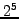components.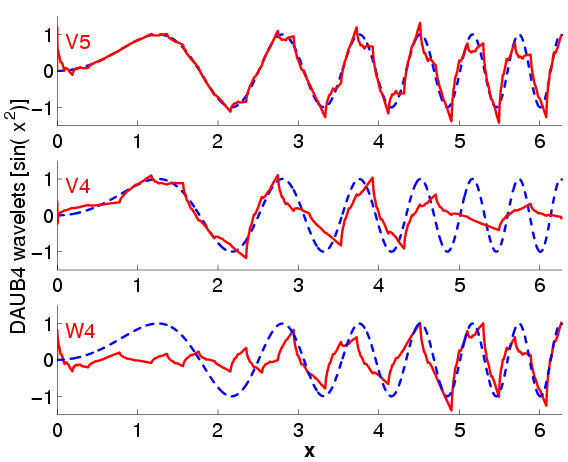Apart from Haar and Daubechies wavelets, a whole family can be generated with a cascading process that is nicely illustrated in the Wavelet Cascade Applet. Wavelets are still relatively new and remain a matter of active research : they have been proven useful in approximation theory, but are still being developed both for differential and integral equations. Clearly apparent in the figures (1.4.6#fig.1) and (1.4.6#fig.2) is the difficulty of incorporating the boundary conditions. Another issue that will become clear in the coming sections, is the difficulty of calculating overlap integrals.

SYLLABUS  Previous: 1.4.5 Harmonic functions  Up: 1.4 Numerical discretization  Next: 1.4.7 Sampling with quasi-particles